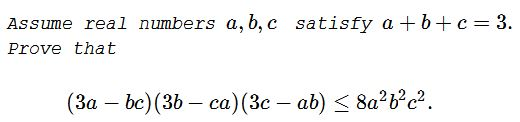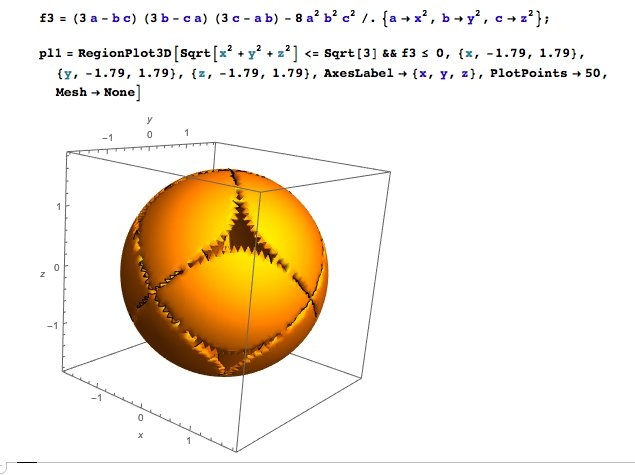An Inequality with Constraint XIII

StatementSolution

Denote $ab+bc+ca=3(1-t^2),\;$ $t\ge 0,\;$ and $abc=r.\;$ After easy calculations, the inequality becomes

$2r(5+t^2)-r^2\le 9(1-t^20^2.$

According to a theorem of Vo Quoc Ba Can, for every $t,\;$ $r\le 2t^3-3t^2+1.$

Consider quadratic polynomial $f(x)=2x(5+t^2)-x^2\;$ on $\mathbb{R}.\;$ Obviously, $f\;$ is strictly increasing on $(-\infty,5++t^2]\;$ and strictly decreasing on $[5+t^2,\infty).\;$ We shall consider two cases.

Case 1: $t^3-3t^2+1\le 5+t^2.$

This is equivalent to $t\in[0,t_0],\;$ where $t_0\gt 2.\;$ We have $r\le 2t^3-3t^2+1\le 5+t^2,\;$ implying $f(r)\le f(2t^3-3t^2+1).\;$ Suffice it to show that $f(2t^3-3t^2+1)\le 9(1-t^2).\;$ But this is equivalent to the obvious $t^2(10t)^4.$

Case 2: $t^3-3t^2+1\gt 5+t^2.$

This is equivalent to $t\gt t_0.\;$ Then $f(r)\le f(5+t^2)=(5+t^2)^2.|;$ Suffice it to show that $(5+t^2)^2\le 9(1-t^2)^2\;$ which is equivalent to $2\le t.$ And this is true since $t\gt t+0\gt 2.\;$ We are done.

Equality is achieved at $(1,1,1)\;$ and the permutations of $(3,0,0).$

Graphical Illustration 1

With some geometry:And "the limt":Emanuel Derman's approach visualized. (My guess: write $a = x^2;\;$ $b=y^2;\;$ $c=z^2.\;$ $x^2+y^2+z^2=3\;$ are points on a sphere of radius $3^0.5.\;$ Now go to polar coordinates and see what the equality says.)

The combination lies inside the sphere:Graphical Illustration 2

Johan Rhodin: Give that RegionPlot3D some more PlotPoints:Acknowledgment

The problem and the Solution have been kindly communicated by Leo Giugiuc. The problem is due to Nguyen Viet Hung; the solution to Leo Giugiuc. The illustration 1 is by Nassim Nicholas Taleb.

Inequalities with the Sum of Variables as a Constraint